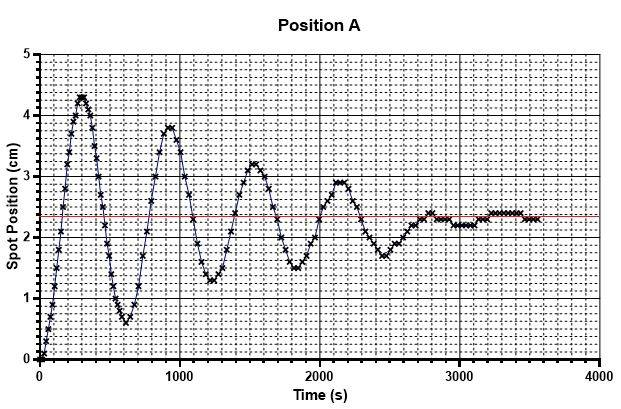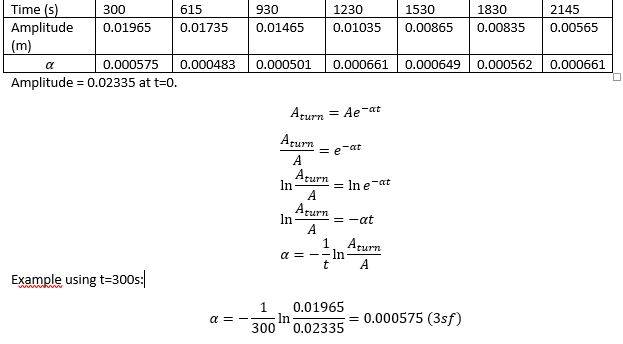Decay coefficient

Homework Statement

How can I estimate the decay coefficient in Ae^-at for this graphI know the equilibrium position

Homework Equations

damped oscillation

The Attempt at a Solutionnot sure if this is right.[/B]

Attachments

•